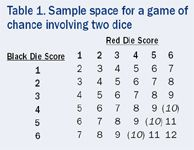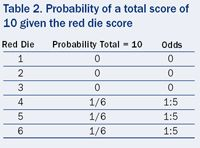# Baseline Balance and Valid Statistical Analyses: Common Misunderstandings

Article

Applied Clinical Trials

Applied Clinical TrialsApplied Clinical Trials-03-01-2005

A simple game of chance clears up the confusion over what randomization can and cannot do.

Recent debates in ACT have shown that random imbalance in clinical trials is a topic of lively controversy.1-3 During my interactions with trialists in industry, academia, and the public health sector, I have come across what I believe are serious misunderstandings regarding what randomization can and cannot do to underwrite the validity of statistical inferences from clinical trials. In this commentary I use games of chance to attempt a simple explanation of the issues.

I believe there are two misunderstood points regarding randomization. First, randomization in clinical trials enables one to deal validly with the effect of unmeasured covariates. In particular, in the absence of any prior information about the effect of treatment and of any knowledge about the actual distribution of covariates in the given trial under question, valid statements may be issued using simple analyses.Table 1. Sample space for a game of chance involving two dice

Second, given knowledge of the baseline distribution, however, such simple statements are not valid and more complex analyses may be called for.

### Two dice-y games of chance

These points will be illustrated using a simple example involving the rolling of two fair dice, a red and a black die. The dice are to be rolled red first, and a statistician is to make a bet regarding the outcome, which involves his correctly calling the odds so that the sum of the scores will be 10. The game is to be played in two different variants. In the first variant, although the dice are rolled in the sequence red then black, the score on neither die is revealed until the statistician has called the odds. The question is, "What odds should he call?" In the second variant, the result of rolling the red die is shown to the statistician before he has to call the odds.

The basic probability setup can be illustrated by Table 1, the "sample space" of all possible outcomes. The table represents the 36 possible combinations of scores from the two dice. The combinations where the total score is 10 are identified by parentheses.

For the first game, the statistician can argue as follows. There are 36 possible combinations of results on the two dice, red = 1 and black = 1 , red = 2 and black= 1, and so forth on to red = 6 and black = 6. Each of these combinations is equally likely, but three of them yield a total of 10. Therefore the probability required is 3/26 = 1/12 and the odds are 1:11.Table 2. Probability of a total score of 10 given the red die score

For the second game the situation is as given in Table 2. If the statistician sees that the result of the first roll is a one, two or three, he can recognize that it is impossible that the total score will be 10. He therefore calls the odds as zero. On the other hand, if the result is four or higher, there is exactly one possible score on the second die that will produce a total of 10. Hence in each of these cases, the probability of a total of 10 is 1/6.

In terms of Table 1, the statistician recognizes that the result must appear in a given row of the table and uses the possible results in that row only to calculate the probability. The statistician's jargon here is that the row constitutes a recognizable subset of the sample space.

According to the great statistician, geneticist, and evolutionary biologist R.A. Fisher (who introduced randomization to the design of agricultural experiments), where such subsets can be recognized they, and not the sample space as a whole, must be used for inference. In fact in his book Statistical Methods and Scientific Inference,4 Fisher considered the case of a gambler throwing a die:

Before the limiting ratio of the whole set can be accepted as applicable to a particular throw, a second condition must be satisfied, namely that before the die is cast no such subset can be recognized.

Note also that the way that we obtained the answer for game two suggests an alternative way of reasoning for game one. The statistician can say to himself, "if I saw the red die, there would be half a chance that the probability of getting 10 would be zero and half a chance it would be 1/6. So, overall, my probability is a half chance of zero and a half chance of 1/6, so the odds are 1:11."

Note that this approach yields exactly the same result for game one as our previous calculation. Averaging over all possible relevant subsets is equivalent to ignoring the existence of such subsets. This behavior is appropriate when the particular subset that applies cannot be recognized, but not otherwise. The result of the red die has not been revealed, therefore there is no recognizable subset and this behavior is therefore reasonable.

### A twelve-sided game of chance

Now consider a third game. A fair 12-sided or dodecahedral die is constructed. One of the 12 faces is marked with a cross. The statistician has to call the odds before the die is rolled. The probability of rolling the cross is clearly 1/12.

Note that from the practical purpose of calling the odds, there is no difference between game three and game one.

### The point of these analogies

The result of the red die is analogous to the distribution at baseline once a clinical trial has been randomized. The total score of the two dice is analogous to the result observed at the end of the trial. Game one corresponds to a trial in which baseline variables are unobserved; game two corresponds to one in which they are observed. Game three corresponds to a trial in which as far as anyone knows, there simply are no prognostic covariates. Not only has the baseline distribution not been measured, it is unmeasurable because no one has any idea what it might be relevant to measure.

### Two common misunderstandings

The first misunderstanding is to treat games one and three differently. The odds that the statistician issues for game one are no less nor more valid than the odds issued for game three. It would be physically possible in principle for the results of the red die to be revealed, but the rules of the game forbid it. Hence, game two is formally equivalent to game three, and there is no point in worrying about what the value of the red die might be. The analogous error for a clinical trial is to query the validity of a correctly calculated treatment estimate and standard error for the case in which prognostic covariates might have been measured, but were not on the grounds that these covariates might be imbalanced. Physicians are particularly liable to commit this error.

The second misunderstanding is to treat game two as if it were game one. Given that the result of the red die has been observed, it is simply incorrect to call the odds that apply to game one. The analogous mistake is to argue that in a clinical trial in which prognostic covariates have been measured, the covariates can be ignored because one has randomized. This is an error to which (naÃ¯ve) frequentist statisticians are particularly liable.

### Practical implications

In my view, there are two implications of an understanding of what randomization can and cannot do in clinical trials.

First, one should not use the fact that one has randomized as an excuse for ignoring baseline prognostic information in analyzing a clinical trial. Such prognostic information provides the means of distinguishing the particular clinical trial from the much larger set of trials one might have run in which the baseline prognostic distribution might have been different.

For example, having observed a baseline difference of 3 mmHg in blood pressure between treatment groups and a difference of 8 mmHg at outcome, the relevant way to calculate the P-value is not to ask, "Given no treatment effect, with what probability would a randomized trial show a difference at outcome of more than 8 mmHg?" Rather, the question should be, "Given no treatment effect, with what probability would a randomized trial with a difference of 3 mmHg at baseline show a difference at outcome of more than 8 mmHg?" An answer to such a question can be provided by using the statistical technique called analysis of covariance.

Also, given that one has taken account of relevant measured prognostic covariates and has randomized, there is no point worrying about the distribution of unmeasured covariates. In the absence of actual distribution in such covariates, the distribution they would have in probability over all randomizations is the appropriate distribution to use. This is analogous to the way that one may use the whole of the possible distribution of scores for the red die if one has not observed which score applies.

A very similar issue arrives in insurance. An insurance company can appropriately set premiums for an individual using the statistics for a large set of similar individuals provided that it cannot be recognized that the individual differs in some way from the average in question. If the individual knows a relevant piece of information, such as the result of a medical or genetic test, then the average risk is no longer applicable.

These simple points may seem so obvious as to be not worth stating. Yet in my opinion, they are often misunderstood. In fact, I was present at a regulatory hearing only a few years ago in which a regulator (not a statistician) argued that the results of a randomization in a double-blind trial were not valid because there was a run-in period after randomization. The regulator also argued that although those baseline values measured at randomization were comparable, the baseline values just prior to treatment might not be. There are several misunderstandings here. First, randomization does not guarantee balance. Second, balanced covariates may not be ignored. Third, the possible distribution of unmeasured covariates in a validly randomized trial does not invalidate the probability statements about the effect of treatment.

Trialists continue to use their randomization as an excuse for ignoring prognostic information, and they continue to worry about the effect of factors they have not measured. Neither practice is logical.

### References

1. M. Buyse and D. McEntegart, "Achieving Balance in Clinical Trials,"

Applied Clinical Trials,

13, 36-40 (May 2004).

2. S.J. Senn, "Unbalanced Claims for Balance," Applied Clinical Trials, 13, 15-16 (June 2004).

3. S. Day, J-M. Grouin, J.A. Lewis, "Achieving Balance in Clinical Trials," Applied Clinical Trials 14, 24-26 (January 2005).

4. R.A. Fisher, Statistical Methods, Experimental Design and Scientific Inference, J.H. Bennet, ed. (Oxford University, Oxford, 1956).

Stephen Senn, PhD, is professor of statistics, Department of Statistics, University of Glasgow, Glasgow G12 8QQ, U.K., email: stephen@stats.gla.ac.uk.